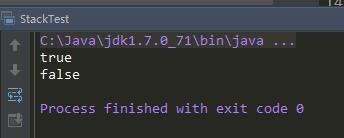Java实现栈和队列面试题

更新时间：2015年09月18日 15:07:27   投稿：lijiao我要评论

（1）栈的创建

（2）队列的创建

（3）两个栈实现一个队列

（4）两个队列实现一个栈

（5）设计含最小函数min()的栈，要求min、push、pop、的时间复杂度都是O(1)

（6）判断栈的push和pop序列是否一致

1、栈的创建：

public class Stack {

public Node current;

//方法：入栈操作
public void push(int data) {
if (head == null) {
head = new Node(data);
} else {
Node node = new Node(data);
node.pre = current;//current结点将作为当前结点的前驱结点
current = node; //让current结点永远指向新添加的那个结点
}
}

public Node pop() {
if (current == null) {
return null;
}

Node node = current; // current结点是我们要出栈的结点
current = current.pre; //每出栈一个结点后，current后退一位
return node;

}

class Node {
int data;
Node pre; //我们需要知道当前结点的前一个结点

public Node(int data) {
this.data = data;
}
}

public static void main(String[] args) {

Stack stack = new Stack();
stack.push(1);
stack.push(2);
stack.push(3);

System.out.println(stack.pop().data);
System.out.println(stack.pop().data);
System.out.println(stack.pop().data);
}

}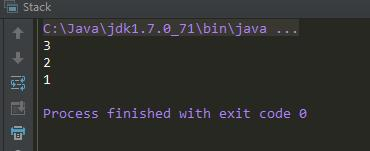2、队列的创建：

public class Queue {
public Node curent;

//方法：链表中添加结点
public void add(int data) {
if (head == null) {
head = new Node(data);
} else {
curent.next = new Node(data);
curent = curent.next;
}
}

//方法：出队操作
public int pop() throws Exception {
if (head == null) {
throw new Exception("队列为空");
}

Node node = head; //node结点就是我们要出队的结点

return node.data;

}

class Node {
int data;
Node next;

public Node(int data) {
this.data = data;
}
}

public static void main(String[] args) throws Exception {
Queue queue = new Queue();
//入队操作
for (int i = 0; i < 5; i++) {
}

//出队操作
System.out.println(queue.pop());
System.out.println(queue.pop());
System.out.println(queue.pop());

}
}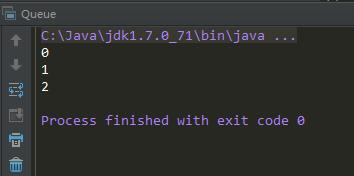3、两个栈实现一个队列：

import java.util.Stack;

/**
* Created by smyhvae on 2015/9/9.
*/
public class Queue {

private Stack<Integer> stack1 = new Stack<>();//执行入队操作的栈
private Stack<Integer> stack2 = new Stack<>();//执行出队操作的栈

//方法：给队列增加一个入队的操作
public void push(int data) {
stack1.push(data);

}

//方法：给队列正价一个出队的操作
public int pop() throws Exception {

if (stack2.empty()) {//stack1中的数据放到stack2之前，先要保证stack2里面是空的（要么一开始就是空的，要么是stack2中的数据出完了），不然出队的顺序会乱的，这一点很容易忘

while (!stack1.empty()) {
stack2.push(stack1.pop());//把stack1中的数据出栈，放到stack2中【核心代码】
}

}

if (stack2.empty()) { //stack2为空时，有两种可能：1、一开始，两个栈的数据都是空的；2、stack2中的数据出完了
throw new Exception("队列为空");
}

return stack2.pop();
}

public static void main(String[] args) throws Exception {
Queue queue = new Queue();
queue.push(1);
queue.push(2);
queue.push(3);

System.out.println(queue.pop());

queue.push(4);

System.out.println(queue.pop());
System.out.println(queue.pop());
System.out.println(queue.pop());

}

}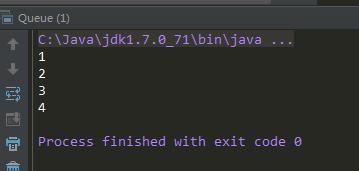4、两个队列实现一个栈：

import java.util.ArrayDeque;
import java.util.Queue;

/**
* Created by smyhvae on 2015/9/9.
*/
public class Stack {

Queue<Integer> queue1 = new ArrayDeque<Integer>();
Queue<Integer> queue2 = new ArrayDeque<Integer>();

//方法：入栈操作
public void push(int data) {
}

//方法：出栈操作
public int pop() throws Exception {
int data;
if (queue1.size() == 0) {
throw new Exception("栈为空");
}

while (queue1.size() != 0) {
if (queue1.size() == 1) {
data = queue1.poll();
while (queue2.size() != 0) { //把queue2中的全部数据放到队列一中
return data;
}
}
}
throw new Exception("栈为空");//不知道这一行的代码是什么意思
}

public static void main(String[] args) throws Exception {
Stack stack = new Stack();

stack.push(1);
stack.push(2);
stack.push(3);

System.out.println(stack.pop());
System.out.println(stack.pop());
stack.push(4);
}
}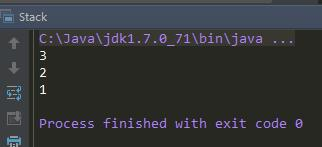5、设计含最小函数min()的栈，要求min、push、pop、的时间复杂度都是O(1)。min方法的作用是：就能返回是栈中的最小值。【微信面试题】

import java.util.Stack;

/**
* Created by smyhvae on 2015/9/9.
*/
public class MinStack {

private Stack<Integer> stack = new Stack<Integer>();
private Stack<Integer> minStack = new Stack<Integer>(); //辅助栈：栈顶永远保存stack中当前的最小的元素

public void push(int data) {
stack.push(data); //直接往栈中添加数据

//在辅助栈中需要做判断
if (minStack.size() == 0 || data < minStack.peek()) {
minStack.push(data);
} else {
}
}

public int pop() throws Exception {
if (stack.size() == 0) {
throw new Exception("栈中为空");
}

int data = stack.pop();
minStack.pop(); //核心代码
return data;
}

public int min() throws Exception {
if (minStack.size() == 0) {
throw new Exception("栈中空了");
}
return minStack.peek();
}

public static void main(String[] args) throws Exception {
MinStack stack = new MinStack();
stack.push(4);
stack.push(3);
stack.push(5);

System.out.println(stack.min());
}
}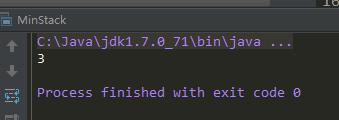6、判断栈的push和pop序列是否一致：

1、2、3、4、5

5、4、3、2、1（正确）

4、5、3、2、1（正确）

4、3、5、1、2（错误）

import java.util.Stack;
/**
* Created by smyhvae on 2015/9/9.
*/
public class StackTest {

//方法：data1数组的顺序表示入栈的顺序。现在判断data2的这种出栈顺序是否正确
public static boolean sequenseIsPop(int[] data1, int[] data2) {
Stack<Integer> stack = new Stack<Integer>(); //这里需要用到辅助栈

for (int i = 0, j = 0; i < data1.length; i++) {
stack.push(data1[i]);

while (stack.size() > 0 && stack.peek() == data2[j]) {
stack.pop();
j++;
}
}
return stack.size() == 0;
}

public static void main(String[] args) {

Stack<Integer> stack = new Stack<Integer>();

int[] data1 = {1, 2, 3, 4, 5};
int[] data2 = {4, 5, 3, 2, 1};
int[] data3 = {4, 5, 2, 3, 1};

System.out.println(sequenseIsPop(data1, data2));
System.out.println(sequenseIsPop(data1, data3));
}
}